# Solution assignment 07 The basics

### Assignment 7

Solve the following equations with the sum-product method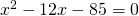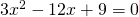### Solution

Just take a look at the rules of the sum-product method and then we can easily find the solutions: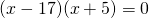, dus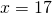of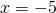For the next assignment we first divide left- and right-hand side by 3. Then we factorize the left-hand side: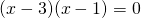, dus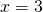of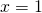We can solve the last assignment by factorizing the left-hand side: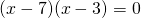, en dus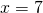of